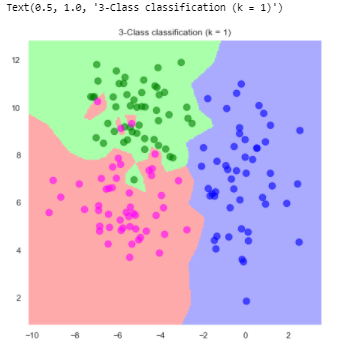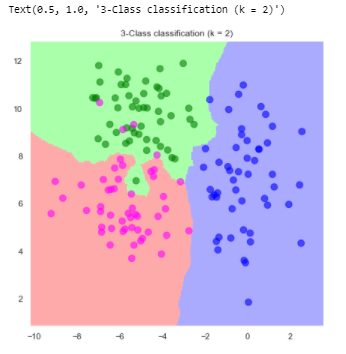Search

# Supervised Machine Learning Algorithms Help |Important Supervised Algorithms

In this blog we have covers all supervised Learning algorithms code implementation, there are many types of supervised learning algorithms as per below:

• K-NN Classification

• Naive Bayes Classifier

• Random Forest

• At last we will learn about "Distance"

#### K-NN Classification

To be able to illustrate how we perform kNN classification in Python, we need some data first. Therefore we synthesize some data from 3 classes. We assume the data in each class comes from a multivariate random distribution.

Import Libraries

```#import Libraries
import numpy as np
import matplotlib.pyplot as plt
import seaborn as sns
%matplotlib inline
sns.set()```

```np.random.seed(100)
n_per_class = 50
colors = ['green', 'blue', 'magenta']

mean1 = [-5, 10]
cov1 = [[1.5, 0], [0, 1.5]]
mean2 = [0, 7]
cov2 = [[1.5, 0], [0, 3]]
mean3 = [-6, 6]
cov3 = [[2, 0], [0, 1.5]]

means = [mean1, mean2, mean3]
covs = [cov1, cov2, cov3]

x11, x12 = np.random.multivariate_normal(means, covs, n_per_class).T
x21, x22 = np.random.multivariate_normal(means, covs, n_per_class).T
x31, x32 = np.random.multivariate_normal(means, covs, n_per_class).T

scale = 75
alpha = 0.6

fig, ax  = plt.subplots(figsize=(7, 7), dpi=300)
ax.scatter(x11, x12, alpha=alpha, color=colors, s=scale)
ax.scatter(x21, x22, alpha=alpha, color=colors, s=scale)
ax.scatter(x31, x32, alpha=alpha, color=colors, s=scale)

ax.set_title("synthesized data for 3 classes")
ax.set_xlabel("\$x_1\$")
ax.set_ylabel("\$x_2\$")```

Output:Then we have to instantiate a kNN classifier from sklearn.

```
from sklearn import neighbors

weights='uniform'
k = 15
knn = neighbors.KNeighborsClassifier(k,weights=weights)

```

We need to pass one array as training features and on array as training labels to the knn object. Therefore we have to put all the attributes together (also class labels).

```x1 = np.r_[x11, x21, x31]
x2 = np.r_[x12, x22, x32]
X_train = np.c_[x1, x2]```

`Y_train = np.r_[0*np.ones(n_per_class), 1*np.ones(n_per_class), 2*np.ones(n_per_class)]`

Now we can fit the model

`knn.fit(X_train, Y_train)`

Now we will see how kNN classifies a point.

```k = 1
knn = neighbors.KNeighborsClassifier(k)
knn.fit(X_train, Y_train)```
```from matplotlib.colors import ListedColormap
cmap_bold = ListedColormap(['green', 'blue', 'magenta'])```

```fig,ax = plt.subplots(figsize=(7, 7))

ax.scatter(X_train[:, 0], X_train[:, 1], c=Y_train, cmap=cmap_bold, alpha=alpha, s=scale)

plt.title("3-Class classification (k = {})".format(k))

X_test = [-7, 10]
Y_pred = knn.predict(X_test)
ax.scatter(X_test, X_test, marker="x", s=scale, lw=2, c='k')

ax.set_title("3-Class classification (k = {})\n Red point is predicted as class {}".format(k, colors[Y_pred.astype(int)]))```

Decision Boundry kNN effectively partitions the feature space into different sets and assigns the same class label to points belonging to the same partition. This partitioning changes as we change k. We illustrate this below. As you see bigger values of k, partition the space more smoothly.

`from matplotlib.colors import ListedColormap`

```k = 15
knn = neighbors.KNeighborsClassifier(k, weights=weights)
knn.fit(X_train, Y_train)

# step size in the mesh
h = 0.05

# Create colour maps
cmap_light = ListedColormap(['#AAFFAA', '#AAAAFF', '#FFAAAA'])
cmap_bold = ListedColormap(['green', 'blue', 'magenta'])

x1_min, x1_max = X_train[:, 0].min() - 1, X_train[:, 0].max() + 1
x2_min, x2_max = X_train[:, 1].min() - 1, X_train[:, 1].max() + 1
xx1, xx2 = np.meshgrid(np.arange(x1_min, x1_max, h), np.arange(x2_min, x2_max, h))

Z = knn.predict(np.c_[xx1.ravel(), xx2.ravel()])

# Put the result into a color plot
Z = Z.reshape(xx1.shape)

fig,ax = plt.subplots(figsize=(7, 7))
ax.pcolormesh(xx1, xx2, Z, cmap=cmap_light)

# Plot also the training points
ax.scatter(X_train[:, 0], X_train[:, 1], c=Y_train, cmap=cmap_bold, alpha=alpha, s=scale)

plt.xlim(xx1.min(), xx1.max())
plt.ylim(xx2.min(), xx2.max())
plt.title("3-Class classification (k = {})".format(k))```

Output:Now we will investigate the effect of 'k' on decision boundaries. Lets train a classifier with k=1 which means we only use the label of the closest point to predict the label of a test point.

```k = 1
knn = neighbors.KNeighborsClassifier(k, weights=weights)
knn.fit(X_train, Y_train)

Z = knn.predict(np.c_[xx1.ravel(), xx2.ravel()])
Z = Z.reshape(xx1.shape)

fig,ax = plt.subplots(figsize=(7, 7))
ax.pcolormesh(xx1, xx2, Z, cmap=cmap_light)

ax.scatter(X_train[:, 0], X_train[:, 1], c=Y_train, cmap=cmap_bold, alpha=alpha, s=scale)

plt.xlim(xx1.min(), xx1.max())
plt.ylim(xx2.min(), xx2.max())
plt.title("3-Class classification (k = {})".format(k))```

Output:```k = 2
knn = neighbors.KNeighborsClassifier(k, weights=weights)
knn.fit(X_train, Y_train)

Z = knn.predict(np.c_[xx1.ravel(), xx2.ravel()])
Z = Z.reshape(xx1.shape)

fig,ax = plt.subplots(figsize=(7, 7))
ax.pcolormesh(xx1, xx2, Z, cmap=cmap_light)

ax.scatter(X_train[:, 0], X_train[:, 1], c=Y_train, cmap=cmap_bold, alpha=alpha, s=scale)

plt.xlim(xx1.min(), xx1.max())
plt.ylim(xx2.min(), xx2.max())
plt.title("3-Class classification (k = {})".format(k))```

Output:```k = 3
knn = neighbors.KNeighborsClassifier(k, weights=weights)
knn.fit(X_train, Y_train)

Z = knn.predict(np.c_[xx1.ravel(), xx2.ravel()])
Z = Z.reshape(xx1.shape)

fig,ax = plt.subplots(figsize=(7, 7))
ax.pcolormesh(xx1, xx2, Z, cmap=cmap_light)

ax.scatter(X_train[:, 0], X_train[:, 1], c=Y_train, cmap=cmap_bold, alpha=alpha, s=scale)

plt.xlim(xx1.min(), xx1.max())
plt.ylim(xx2.min(), xx2.max())
plt.title("3-Class classification (k = {})".format(k))```

Output:```k = 5
knn = neighbors.KNeighborsClassifier(k, weights=weights)
knn.fit(X_train, Y_train)

Z = knn.predict(np.c_[xx1.ravel(), xx2.ravel()])
Z = Z.reshape(xx1.shape)

fig,ax = plt.subplots(figsize=(7, 7))
ax.pcolormesh(xx1, xx2, Z, cmap=cmap_light)

ax.scatter(X_train[:, 0], X_train[:, 1], c=Y_train, cmap=cmap_bold, alpha=alpha, s=scale)

plt.xlim(xx1.min(), xx1.max())
plt.ylim(xx2.min(), xx2.max())
plt.title("3-Class classification (k = {})".format(k))```

Output:Prediction

```k = 5
knn = neighbors.KNeighborsClassifier(k, weights=weights)
knn.fit(X_train, Y_train)

Z = knn.predict(np.c_[xx1.ravel(), xx2.ravel()])
Z = Z.reshape(xx1.shape)

fig,ax = plt.subplots(figsize=(7, 7))
ax.pcolormesh(xx1, xx2, Z, cmap=cmap_light)

ax.scatter(X_train[:, 0], X_train[:, 1], c=Y_train, cmap=cmap_bold, alpha=alpha, s=scale)

plt.xlim(xx1.min(), xx1.max())
plt.ylim(xx2.min(), xx2.max())
plt.title("3-Class classification (k = {})".format(k))

X_test = [-4, 8]
Y_pred = knn.predict(X_test)
ax.scatter(X_test, X_test, alpha=0.95, color='r', s=3*scale)

ax.set_title("3-Class classification (k = {})\n Red point is predicted as class {}".format(k, colors[Y_pred.astype(int)]))```

Now instead of predicting the class label for one point, we use our model to predict the labels of multiple points.

First we generate some test data from the first class. This way we know the true class labels. Then we can use the kNN classifier to predict labels for the test data and get the predicted class labels. A measure of accuracy for the classifier can be defined by comparing the true and predicted labels.

```n_test = 100
X1_test, X2_test = np.random.multivariate_normal(mean1, cov1, n_test).T
Y_true = 0 * np.ones(n_test)
X_test = np.c_[X1_test, X2_test]
Y_pred = knn.predict(X_test)
Y_pred
Y_pred == Y_true
(sum(Y_pred == Y_true) + 0.0) / n_test```